# PSAT Math : How to find the common difference in sequences

## Example Questions

### Example Question #1 : Sequences

How many integers in the following infinite series are positive: 100, 91, 82, 73 . . . ?

9

13

11

12

10

12

Explanation:

The difference between each number in the series is 9. You can substract nine 11 times from 100 to get 1: 100 – 9x11 = 1. Counting 100, there are 12 positive numbers in the series.

### Example Question #1 : How To Find The Common Difference In Sequences

In a sequence of numbers, each term istimes larger than the one before it. If the 3rd term of the sequence is 12, and the 6th term is 96, what is the sum of all of the terms less than 250?

372

381

378

192

384

381

Explanation:

Let's call the first term in the sequence a1 and the nth term an

We are told that each term is r times larger than the one before it. Thus, we can find the next term in the sequence by multiplying by r.

a1 = a1

a2 = r(a1)

a3 = r(a2) = r(r(a1)) = r2(a1)

a4 = r(a3) = r(r2(a1)) = r3(a1)

an = r(n–1)a1

We can use this information to find r.

The problem gives us the value of the third and the sixth terms.

a3 = r2(a1) = 12

a6 = r5(a1) = 96

Let's solve for a1 in terms of r and a3

a1 = 12/(r2)

Let's then solve for a1 in terms of r and a6.

a1 = 96/(r5)

Now, we can set both values equal and solve for r.

12/(r2) = 96/(r5)

Multiply both sides by r5 to get rid of the fraction.

12r5/r2 = 96

Apply the property of exponents which states that ab/ac = ab–c.

12r3 = 96

Divide by 12 on both sides.

r3 = 8

Take the cube root of both sides.

r = 2

This means that each term is two times larger than the one before it, or that each term is one half as large as the one after it.

a2 must equal a3 divided by 2, which equals 12/2 = 6.

a1 must equal a2 divided by 2, which equals 6/2 = 3.

Here are the first eight terms of the sequence:

3, 6, 12, 24, 48, 96, 192, 384

The question asks us to find the sum of all the terms less than 250. Only the first seven terms are less than 250. Thus the sum is equal to the following:

sum = 3 + 6 + 12 + 24 + 48 + 96 + 192 = 381

### Example Question #1 : How To Find The Common Difference In Sequences

The first term of an arithmetic sequence is 20; the tenth term is 100. What is the sixteenth term?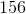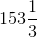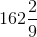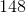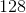Explanation:

Given terms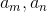of an arithmetic sequence,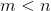, the common differenceis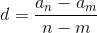Setting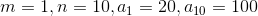, the common difference is found to be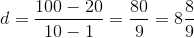Theterm of the sequence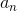is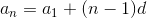Setting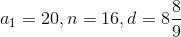, this term is found to be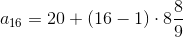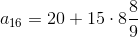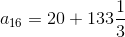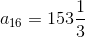### Example Question #2 : How To Find The Common Difference In Sequences

An arithmetic sequence has as its first term 17. Its tenth term is. What is the sum of its second and ninth terms?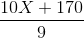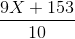None of the other choices gives the correct response.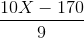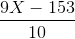None of the other choices gives the correct response.

Explanation:

It is not necessary to determine the common difference or any other terms to answer this question. If we letbe the common difference, then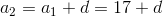and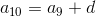, or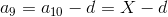The sum of the second and ninth terms is given by the expression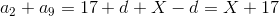### Example Question #3 : How To Find The Common Difference In Sequences

The first and fourth terms of an arithmetic sequence are, respectively,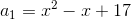and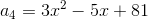.

Which of the following is the common difference of the sequence?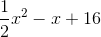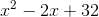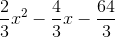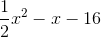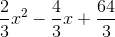Explanation:

Given termsof an arithmetic sequence,, the common differenceis.

Set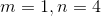: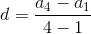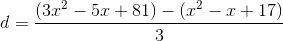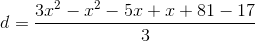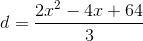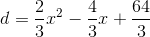### Example Question #3 : How To Find The Common Difference In Sequences

Which of the following are not natural numbers?

I. 1

II. 0

III. 349010

IV. -2

V. 1/4

II, III, IV, V

II, IV, V

I, IV, V

I, V

IV, V